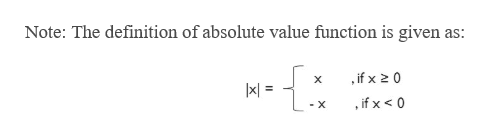Graph the given equation. First plot the solutions of the equation for the given values of x, and then connect the points with a smooth graph.y = |x + 6|x = −9, −8, −7, −6, −5, −4, −3

Question

Graph the given equation. First plot the solutions of the equation for the given values of x, and then connect the points with a smooth graph.

 y = |x + 6| x = −9, −8, −7, −6, −5, −4, −3
Step 1

To plot the graph for |x +6|help_outlineImage TranscriptioncloseNote: The definition of absolute value function is given as: if x 2 0 if x < 0 - X fullscreen
Step 2

Using the definition, we get the val...

Want to see the full answer?

See Solution

Want to see this answer and more?

Our solutions are written by experts, many with advanced degrees, and available 24/7

See Solution
Tagged in

Other